## 5-3 嚙踝蕭嚙踝蕭嚙踝蕭嚙踝蕭嚙踝蕭嚙踝蕭嚙踝蕭嚙踝蕭嚙踝蕭嚙踝蕭嚙踝蕭嚙踝蕭嚙踝蕭嚙踝蕭嚙踝蕭

MATLAB 的 spy 指令可用於觀看稀疏矩陣的非零元素分佈情況，例如：

Example 1: 05-稀疏矩陣/spy01.mload west0479.mat % 載入二進位制檔案 west0479.mat spy(west0479) % 觀看稀疏矩陣的非零元素分佈情況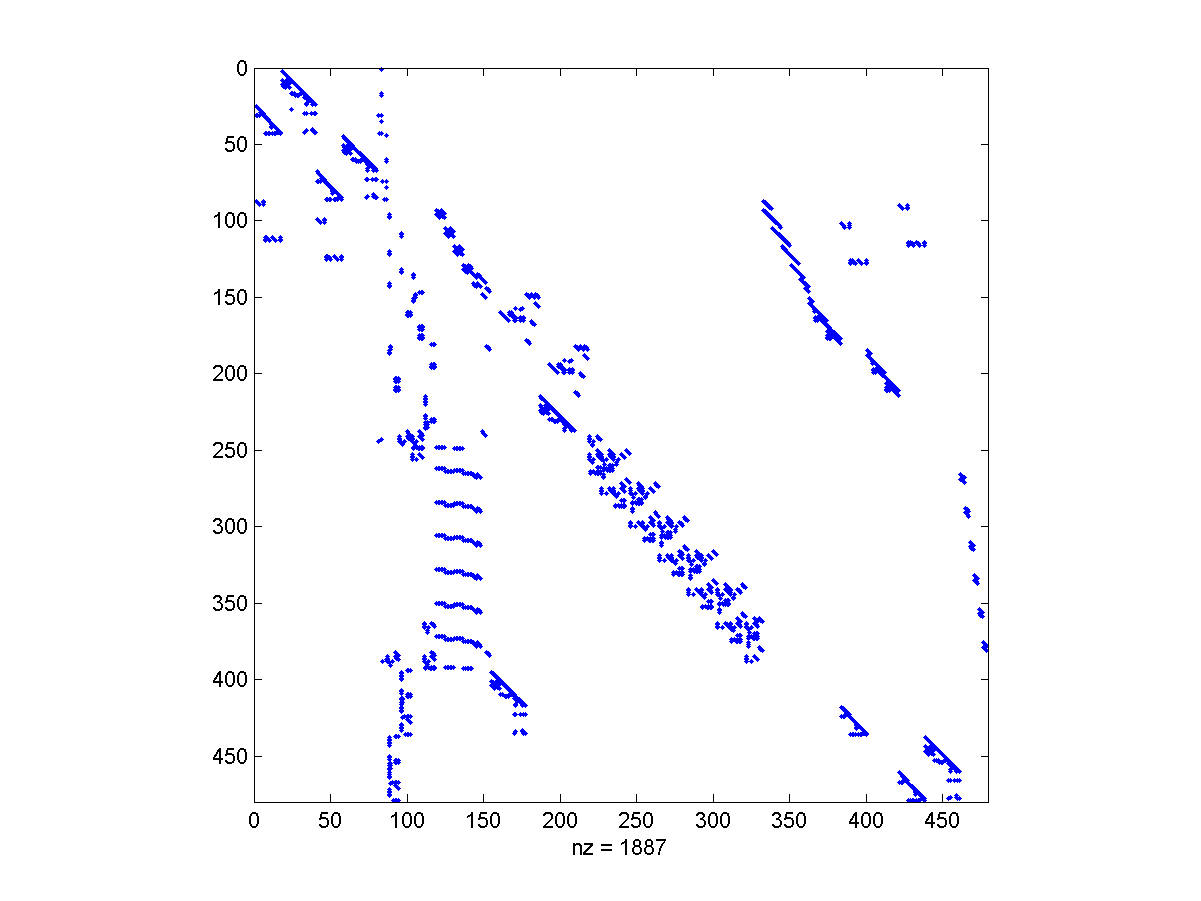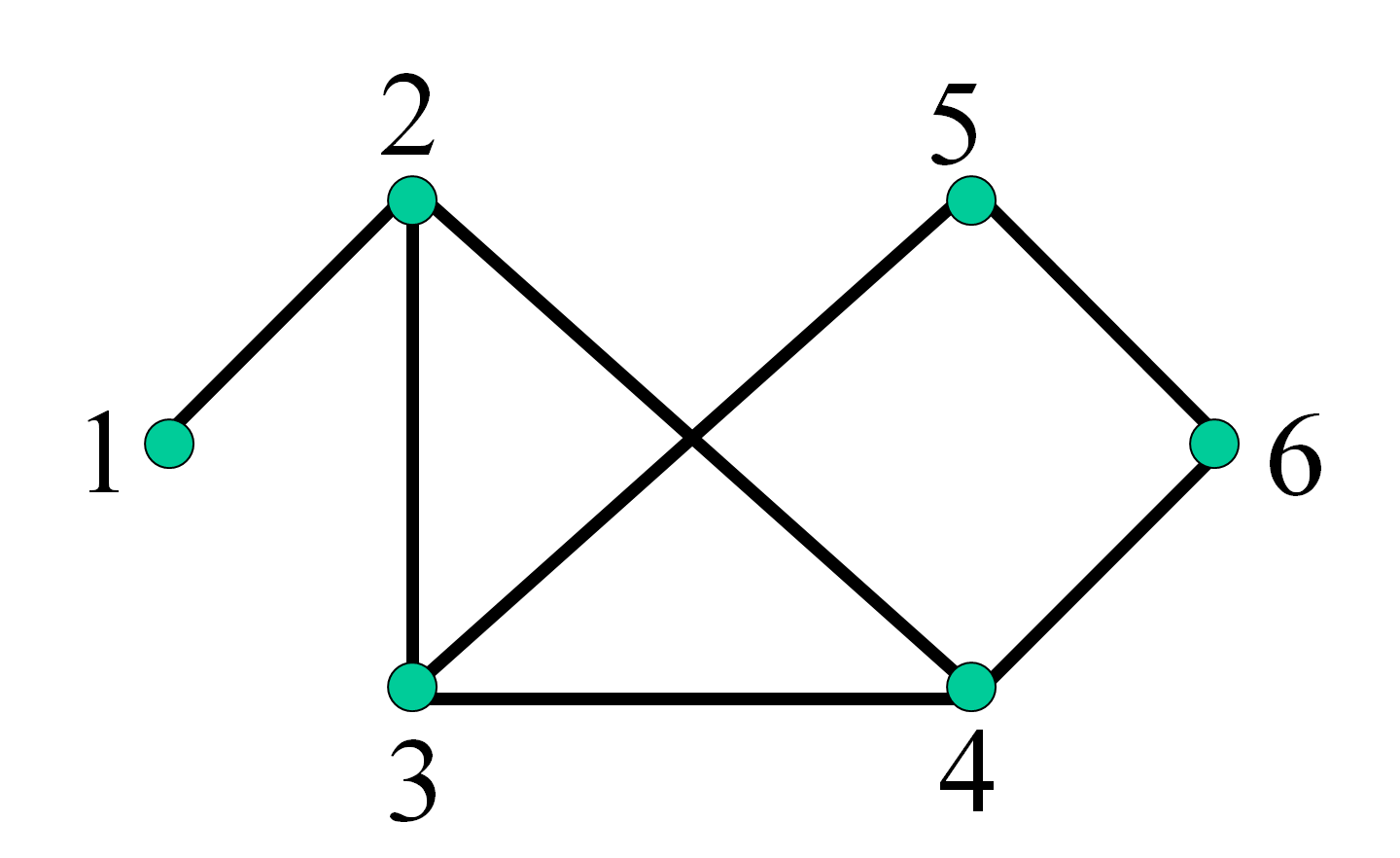>> A = spconvert([1 2 1; 2 3 1; 2 4 1; 3 2 1; 3 4 1; 3 5 1; 4 2 1; 4 3 1; 4 6 1; 5 3 1; 5 6 1; 6 4 1; 6 5 1]) A = 　(1,2)　　　　1 　(3,2)　　　　1 　(4,2)　　　　1 　(2,3)　　　　1 　(4,3)　　　　1 　(5,3)　　　　1 　(2,4)　　　　1 　(3,4)　　　　1 　(6,4)　　　　1 　(3,5)　　　　1 　(6,5)　　　　1 　(4,6)　　　　1 　(5,6)　　　　1

Example 2: 05-稀疏矩陣/gplot01.mA = spconvert([1 2 1; 2 3 1; 2 4 1; 3 4 1; 3 5 1; 5 6 1; 4 6 1]); xy = [0 1; 1 2; 1 0; 2 0; 2 2; 3 1]; % 每一個列向量是一組 (x, y) 座標 gplot(A, xy, '-o') % 畫出無向圖（Undirected Graph）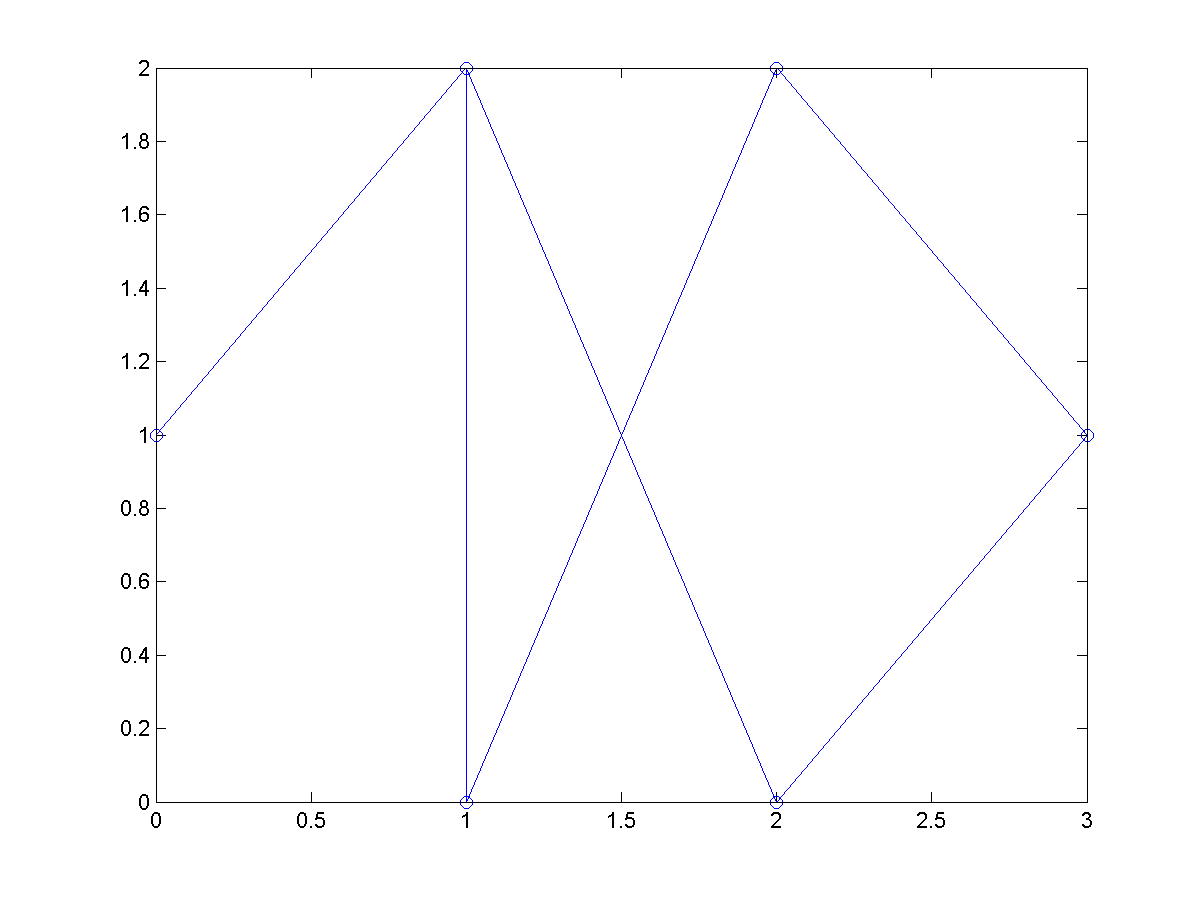Example 3: 05-稀疏矩陣/gplot02.m[A,xy] = bucky; % A 為鄰近矩陣，xy 為座標 gplot(A, xy, '-o'); % 畫出無向圖（Undirected Graph） axis equal % 設定 x 軸和 y 軸的刻度一樣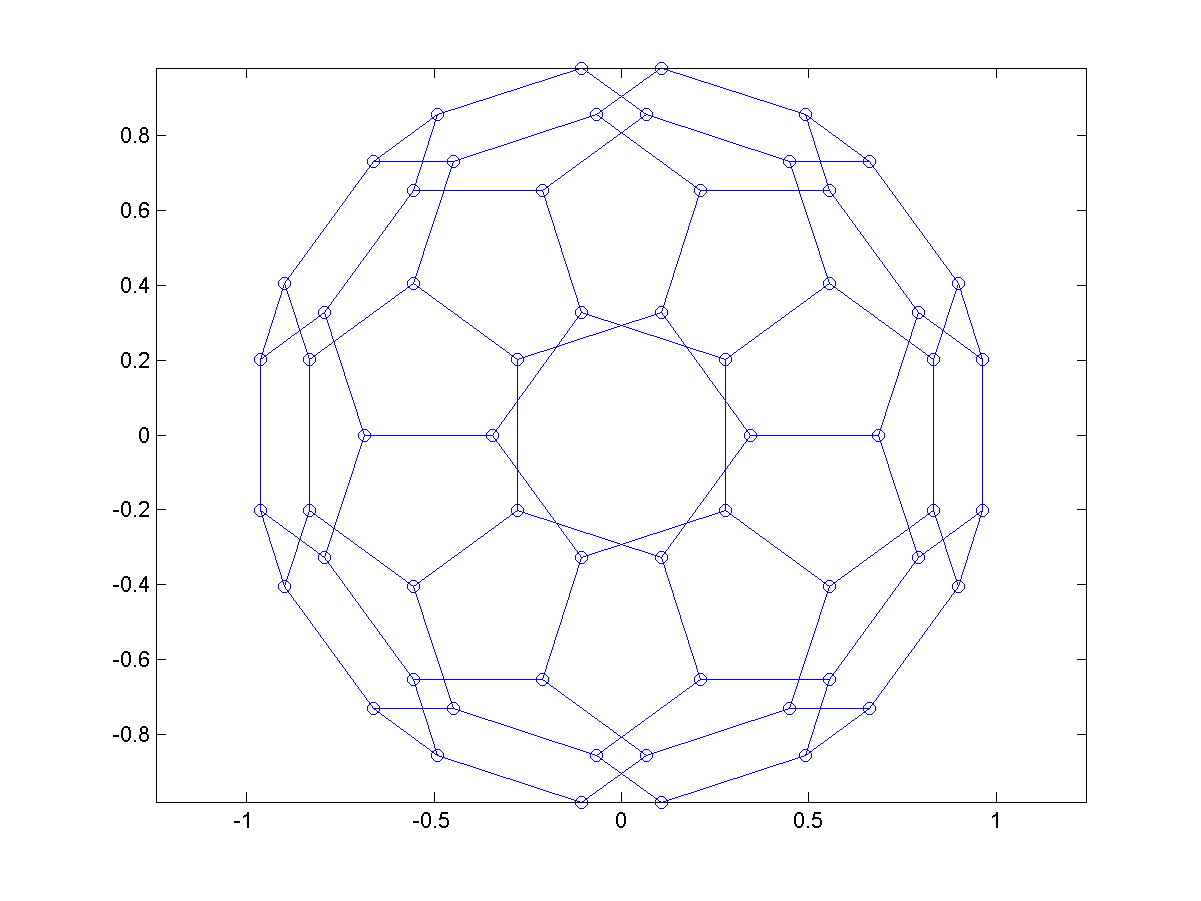Example 4: 05-稀疏矩陣/treePlot01.mnodes = [0 1 2 2 4 4 4 1 8 8 10 10 11 11 11 11]; treeplot(nodes)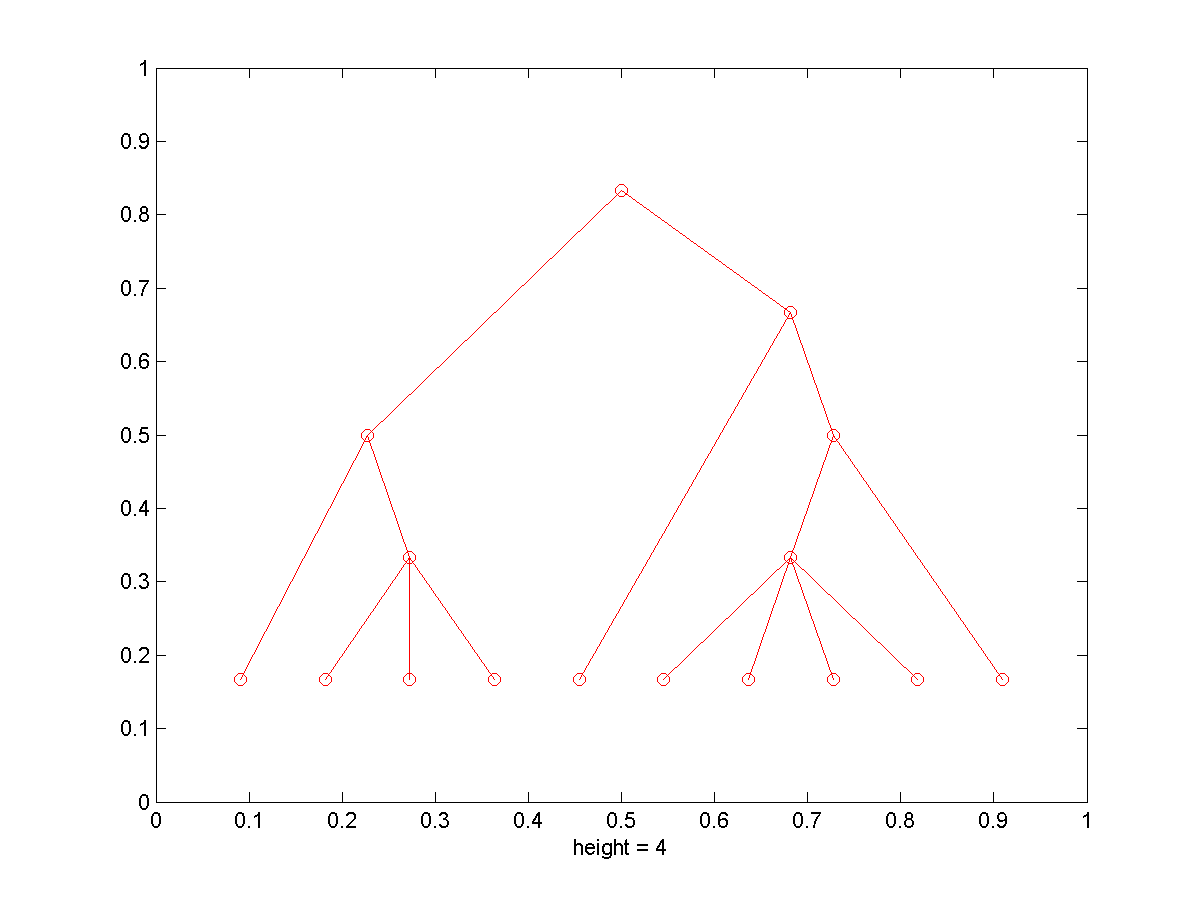Example 5: 05-稀疏矩陣/treePlot02.mnodes = [0 1 2 2 4 4 4 1 8 8 10 10 11 11 11 11]; treeplot(nodes); h=findobj(0, 'type', 'line'); % h(1) is links; h(2) is nodes. xdata=get(h(2), 'xdata'); ydata=get(h(2), 'ydata'); for i=1:length(nodes) text(xdata(i), ydata(i), [' ', int2str(i)]); end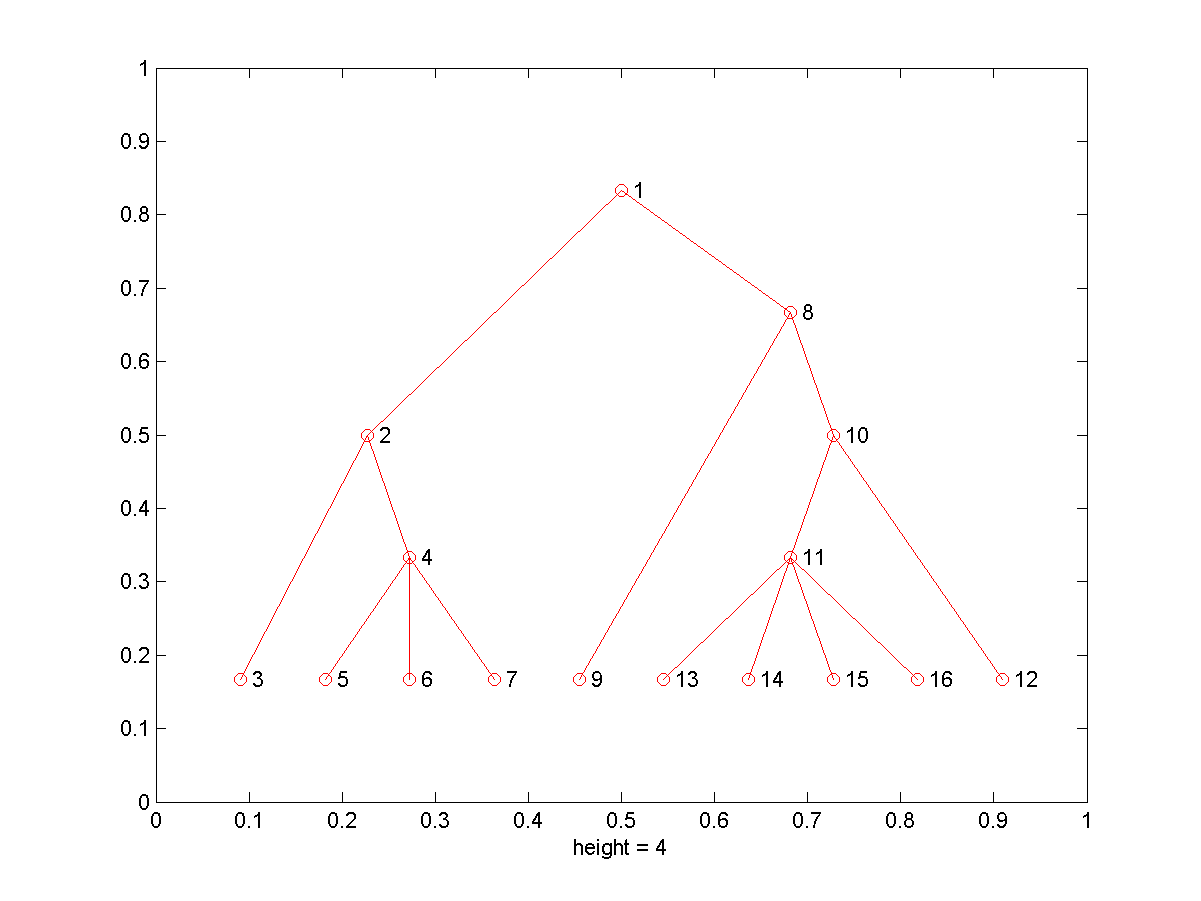MATLAB程式設計：進階篇